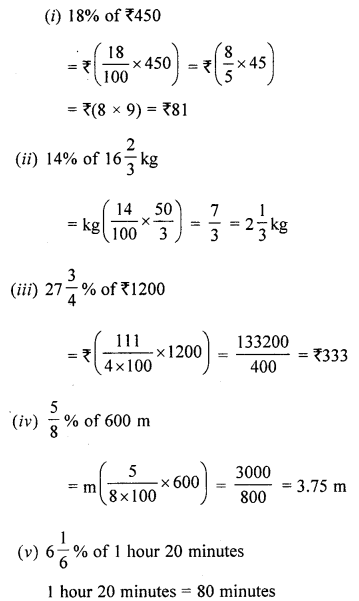## ML Aggarwal Class 6 Solutions for ICSE Maths Chapter 8 Ratio and Proportion Ex 8.4

Question 1.
Find the value of:Solution:Question 2.
In a class of 60 student, 45% are girls. Find the number of boys in the class.
Solution:
Total student = 60
% of girls = 45%
No. of boys = ?
No. of girls = $$60 \times \frac{45}{100}=\frac{6 \times 45}{10}=27$$ girls
No. of boys = Total students – No. of girls = 60 – 27 = 33 boys

Question 3.
Mr. Malkani saves 22% of his salary every month. If his salary is ₹ 12750 per month, what is his expenditure?
Solution:
Total salary = ₹ 12750
Saving = 22%
∴ Total savings = 22% of ₹ 12750
= ₹ 12750 × $$\frac{22}{100}$$ = ₹2805
∴ Total expenditure = ₹ 12750 – ₹2805 = ₹9945

Question 4.
On a rainy day, 94% of the students were present in a school, if the number of students absent on that day was 174, find the total strength of the school.
Solution:
Total % age of students = 100
Student present = 94%
Students absent = (100- 94) = 6%
Let, the total number of students in school = x
$$6 \% \text { of } x=174 \Rightarrow \frac{6}{100} \times x=174$$
$$\Rightarrow x=174 \times \frac{100}{6} \Rightarrow x=29 \times 100=2900$$
∴ Total strength of the school = 2900